Win up to 100% scholarship on Aakash BYJU'S JEE/NEET courses with ABNAT Win up to 100% scholarship on Aakash BYJU'S JEE/NEET courses with ABNAT

# JEE Main 2022 July 25 – Shift 2 Chemistry Question Paper with Solutions

The JEE Main 2022 July 25 – Shift 2 Chemistry Question Paper with Solutions is available on this page. Students can use the JEE Main 2022 answer keys to self-analyse their performance in the JEE Main 2022 exam. JEE Main 2022 question papers with solutions are explained in a detailed manner so that students can easily grasp the concepts used in solving the problems. Students can revise and practise the JEE Main 2022 July 25 – Shift 2 Chemistry Question Paper with Solutions and thus score more marks in the upcoming engineering entrance exams.

## JEE Main 2022 25th July Shift 2 Chemistry Question Paper and Solutions

SECTION – A

Multiple Choice Questions: This section contains 20 multiple choice questions. Each question has 4 choices (1), (2), (3) and (4), out of which ONLY ONE is correct.

1. Match List-I with List-II :

 List-I (Molecule) List-II (hybridization ; shape) A. XeO3 I. sp3d; linear B. XeF2 II. sp3; pyramidal C. XeOF4 III. sp3d3; distorted octahedral D. XeF6 IV. sp3d2; square pyramidal

Choose the correct answer from the options given below:

(A) A-II, B-I, C-IV, D-III

(B) A-II, B-IV, C-III, D-I

(C) A-IV, B-II, C-III, D-I

(D) A-IV, B-II, C-I, D-III

Sol. XeO3sp3 , Pyramidal

XeF_2 — sp3d, linear

XeOF4sp3d2, Square Pyramidal

XeF6sp3d3, distorted octahedral

2. Two solutions A and B are prepared by dissolving 1 g of non-volatile solutes X and Y, respectively in 1 kg of water. The ratio of depression in freezing points for A and B is found to be 1 : 4. The ratio of molar masses of X and Y is

(A) 1 : 4

(B) 1 : 0.25

(C) 1 : 0.20

(D) 1 : 5

Sol. ΔTf = i kf × m

$$\begin{array}{l}\frac{\Delta T_{f(A)}}{\Delta T_{f(B)}}=\frac{1}{4}\end{array}$$
$$\begin{array}{l}\frac{i\times K_f \times \frac{1}{M_A}\times 1}{i\times K_f \times \frac{1}{M_B}\times 1 }=\frac{1}{4}\end{array}$$
$$\begin{array}{l}\frac{M_B}{M_A}=\frac{1}{4}\end{array}$$
$$\begin{array}{l}M_A~\colon M_B = 4~\colon1\end{array}$$

3. Ka1, Ka2, and Ka3 are the respective ionization constants for the following reactions (a), (b), and (c).

$$\begin{array}{l}(a)\ \ H_2C_2O_4\rightleftharpoons H^+ + HC_2O_4^-\end{array}$$
$$\begin{array}{l}(b)\ \ H_2C_2O_4^-\rightleftharpoons H^+ + C_2O_4^{2-}\end{array}$$
$$\begin{array}{l}(c)\ \ H_2C_2O_4 \rightleftharpoons 2H^+ + C_2O_4^{2-}\end{array}$$

The relationship between Ka1, Ka2, and Ka3 is given as

$$\begin{array}{l}(\text{A})\ K_{a_3} = K_{a_1}+K{a_2}\end{array}$$
$$\begin{array}{l}(\text{B})\ K_{a_3} = K_{a_1}-K{a_2}\end{array}$$
$$\begin{array}{l}(\text{C})\ K_{a_3} = K_{a_1}/K{a_2}\end{array}$$
$$\begin{array}{l}(\text{D})\ K_{a_3} = K_{a_1}\times K{a_2}\end{array}$$

Sol.

$$\begin{array}{l}H_2C_2O_4 \rightleftharpoons 2H^+ + C_2O_4^{2-}\ \ \ K_{a_3}\end{array}$$
$$\begin{array}{l}H_2C_2O_4\rightleftharpoons H^+ + HC_2O_4^- \ \ \ K_{a_1}\end{array}$$
$$\begin{array}{l}HC_2O_4^-\rightleftharpoons H^+ + C_2O_4^{2-} \ \ \ K_{a_2}\end{array}$$
$$\begin{array}{l}K_{a_3}=\frac{[H^+]^2[C_2O_{4}^{2-}]}{[H_2C_2O_4]}\end{array}$$
$$\begin{array}{l}K_{a_1}=\frac{[H^+][HC_2O_{4}^{-}]}{[H_2C_2O_4]},K_{a_2}=\frac{[H^+][C_2O_{4}^{-}]}{[HC_2O_4]}\end{array}$$
$$\begin{array}{l}K_{a_3}=K_{a_1} \times K_{a_2}\end{array}$$

4. The molar conductivity of a conductivity cell filled with 10 moles of 20 mL NaCl solution is

$$\begin{array}{l}\Lambda_{m_1}\ \text{and that of 20 moles another identical cell having 80 mL NaCl solution is}\ \Lambda_{m_2}.\end{array}$$
The conductivities exhibited by these two cells are the same. The relationship between
$$\begin{array}{l}\Lambda_{m_2}\ \text{and}\ \Lambda_{m_1}\ \text{is}\end{array}$$

$$\begin{array}{l}(\text{A})\ \Lambda_{m_2}=2\Lambda_{m_1}\end{array}$$
$$\begin{array}{l}(\text{B})\ \Lambda_{m_2}=\Lambda_{m_1}/2\end{array}$$
$$\begin{array}{l}(\text{C})\ \Lambda_{m_2}=\Lambda_{m_1}\end{array}$$
$$\begin{array}{l}(\text{D})\ \Lambda_{m_2}=4\Lambda_{m_1}\end{array}$$

Sol.

$$\begin{array}{l}\Lambda_{m_1}=\frac{k_1\times 1000}{M_1}=\frac{k\times1000}{\frac{10}{0.02}}\end{array}$$
$$\begin{array}{l}\Lambda_{m_2}=\frac{k_2\times 1000}{\frac{20}{0.08}}\end{array}$$

It is given that k1 = k2

$$\begin{array}{l}k_1=\frac{\Lambda_{m_1}}{2}\end{array}$$
$$\begin{array}{l}k_2=\frac{\Lambda_{m_2}}{4}\end{array}$$

Applying the given condition on conductivity.

$$\begin{array}{l}\frac{\Lambda_{m_1}}{2}=\frac{\Lambda_{m_2}}{4}\end{array}$$
$$\begin{array}{l}\Lambda_{m_2}=2\Lambda_{m_1}\end{array}$$

5. For micelle formation, which of the following statements are correct?

A. Micelle formation is an exothermic process.

B. Micelle formation is an endothermic process.

C. The entropy change is positive

D. The entropy change is negative

(A) A and D only

(B) A and C only

(C) B and C only

(D) B and D only

Sol. Micelle formation is an endothermic process with positive entropy change.

6. The first ionization enthalpies of Be, B, N and O follow the order

(A) O < N < B < Be

(B) Be < B < N < O

(C) B < Be < N < O

(D) B < Be < O < N

Sol The first ionisation energy increase from left to right along 2nd period with the following exceptions

IE1 : Be > B and N > O

This is due to stable configuration of Be in comparison to B and that of N in comparison to O.

Hence the correct order is N > O > Be > B.

7. Given below are two statements.

Statement-I : Pig iron is obtained by heating cast iron with scrap iron.

Statement-II : Pig iron has a relatively lower carbon content than that of cast iron.

In the light of the above statements, choose the correct answer from the options given below.

(A) Both Statement-I and Statement-II are correct

(B) Both Statement-I and Statement-II are not correct.

(C) Statement-I is correct but Statement-II is not correct

(D) Statement-I is not correct but Statement-II is correct

Sol Cast iron is made by melting pig iron with scrap iron and coke using hot air blast.

Hence Statement-I is incorrect

But Pig iron has relatively more carbon content

Hence statement-II is incorrect.

8. High purity (>99.95%) dihydrogen is obtained by

(A) Reaction of zinc with aqueous alkali

(B) Electrolysis of acidified water using platinum electrodes

(C) Electrolysis of warm aqueous barium hydroxide solution between nickel electrodes

(D) Reaction of zinc with dilute acid

Sol High purity (>99.95%) H2 is obtained by electrolysing warm aqueous Ba(OH)2 solution between nickel electrodes.

9. The correct order of density is

(A) Be > Mg > Ca > Sr

(B) Sr > Ca > Mg > Be

(C) Sr > Be > Mg > Ca

(D) Be > Sr > Mg > Ca

Sol Density of Sr = 2.63 g/cm3

Density of Be = 1.84 g/cm3

Density of Mg = 1.74 g/cm3

Density of Ca = 1.55 g/cm3

10. The total number of acidic oxides from the following list is

NO, N2O, B2O3, N2O5, CO, SO3, P4O10

(A) 3

(B) 4

(C) 5

(D) 6

Sol NO, N2O, CO – neutral oxides

B2O3, N2O5, SO3, P4O10 – acidic oxides

11. The correct order of energy of absorption for the following metal complexes is

A : [Ni(en)3]2+, B : [Ni(NH3)6]2+, C : [Ni(H2O)6]2+

(A) C < B < A

(B) B < C < A

(C) C < A < B

(D) A < C < B

Sol. Stronger is ligand attached to metal ion, greater will be the splitting between t2g and eg (hence greater will be ΔU), ∴ greater will be absorption of energy.

Hence correct order

[Ni(en)3]2+ > [Ni(NH3)6]2+ > [Ni(H2O)6]2+

12. Match List I with List II.

 List I List II A. Sulphate I. Pesticide B. Fluoride II. Bending of bones C. Nicotine III. Laxative effect D. Sodium arsinite IV. Herbicide

Choose the correct answer from the options given below:

(A) A-II, B-III, C-IV, D-I

(B) A-IV, B-III, C-II, D-I

(C) A-III, B-II, C-I, D-IV

(D) A-III, B-II, C-IV, D-I

Sol. Sodium arsinite — Herbicide

Nicotine — Pesticide

Sulphate — Laxative effect

Fluoride — Bending of bones

13. Major product of the following reaction is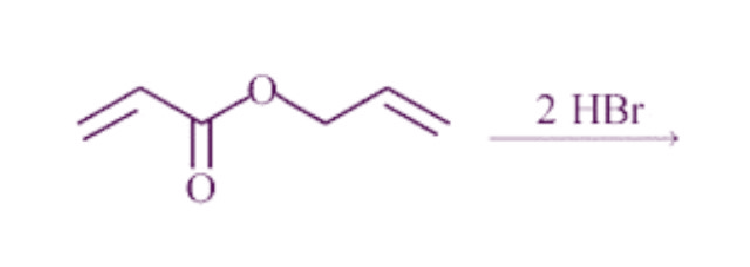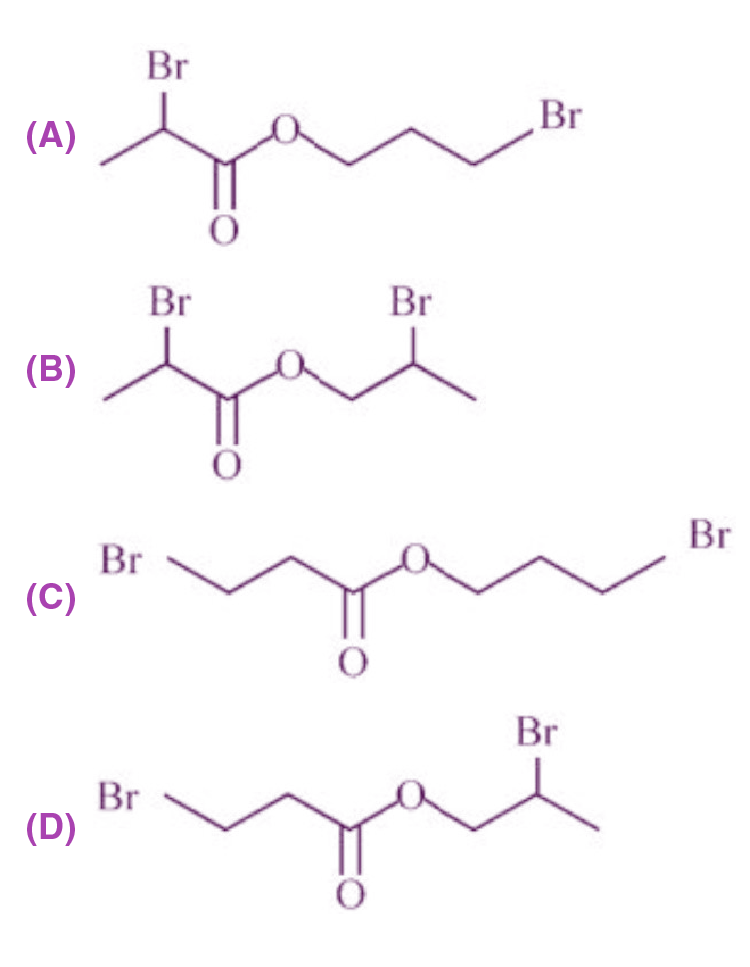Sol.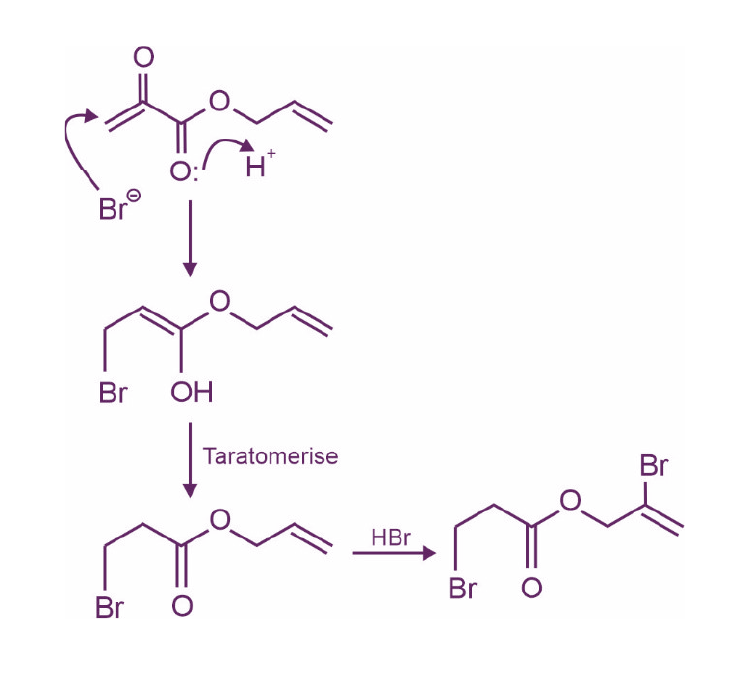14. What is the major product of the following reaction?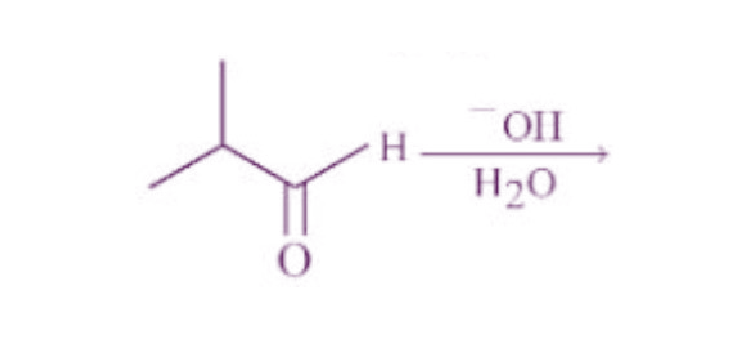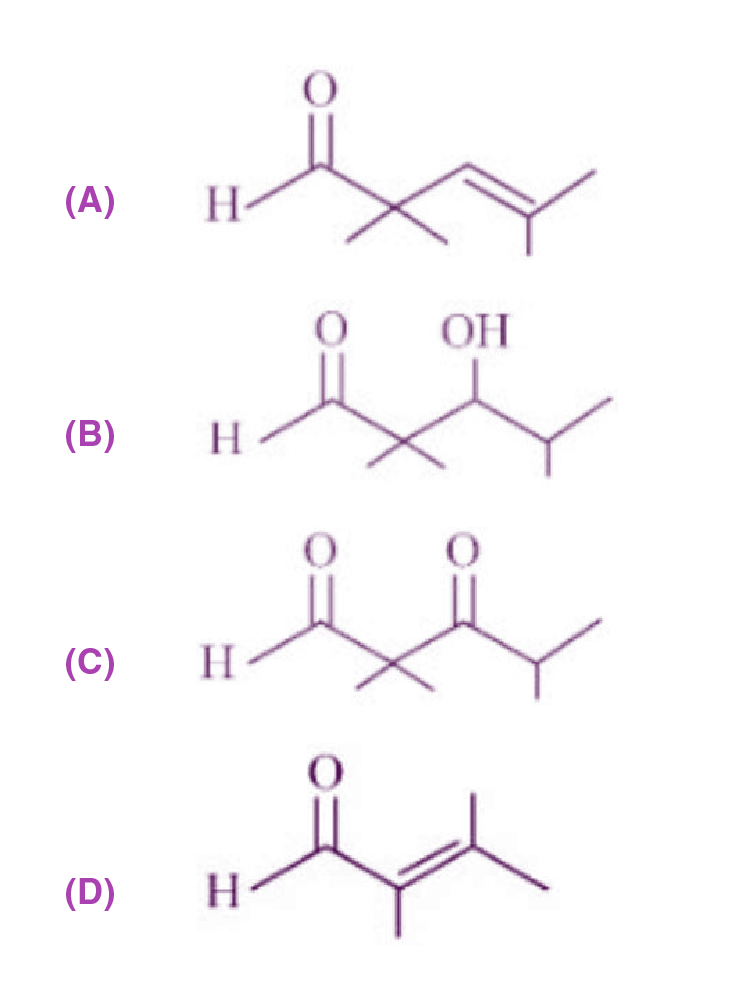Sol.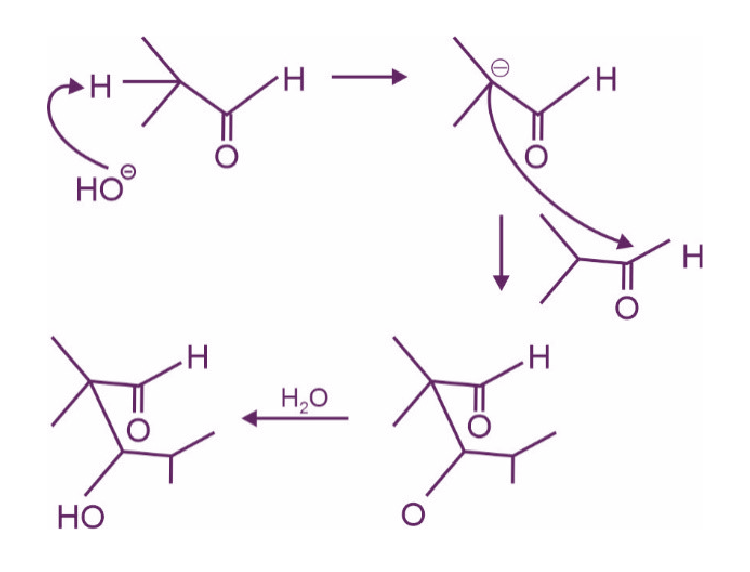15. Arrange the following in decreasing acidic strength.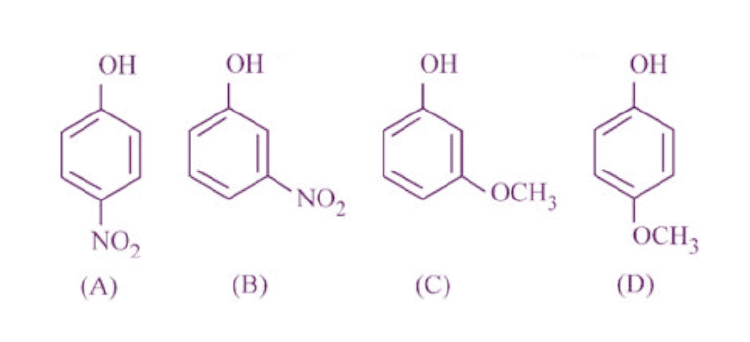(A) A > B > C > D

(B) B > A > C > D

(C) D > C > A > B

(D) D > C > B > A

Sol.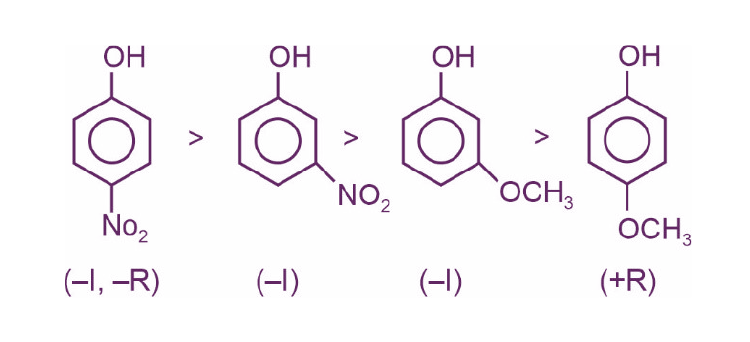16.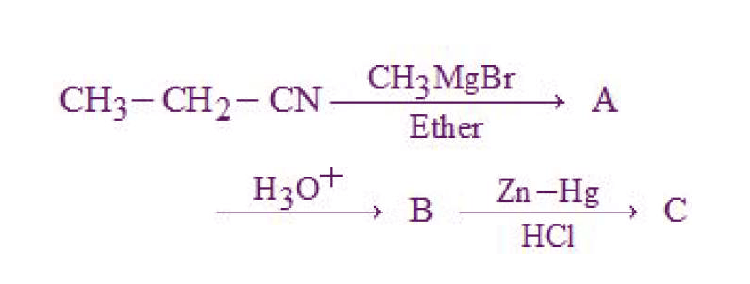The correct structure of C is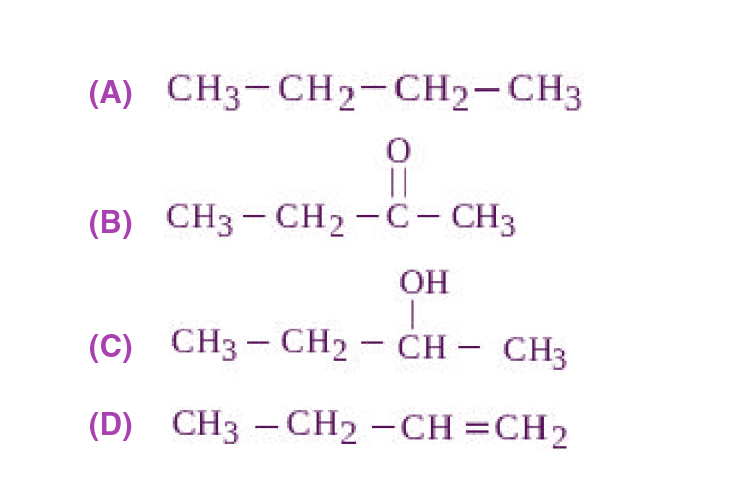Sol.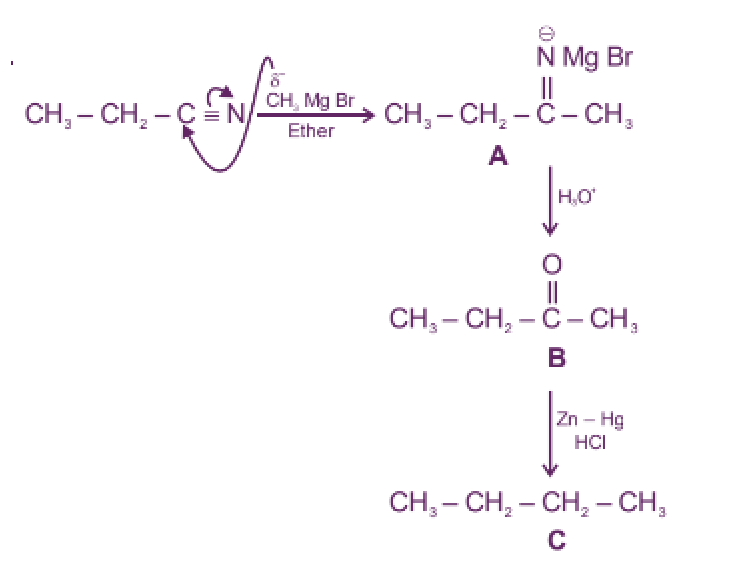17. Match List I with List II:

 List I Polymer List II Used for items A. Nylon 6, 6 I. Buckets B. Low density polythene II. Non-stick utensils C. High densityvpolythene III. Bristles of brushes D. Teflon IV. Toys

Choose the correct answer from the options given below:

(A) A-III, B-I, C-IV, D-II

(B) A-III, B-IV, C-I, D-II

(C) A-II, B-I, C-IV, D-III

(D) A-II, B-IV, C-I, D-III

Sol. Nylon 6, 6 → used in making bristles of brushes

Low density polythene → used in making Toys

High density polythene → used in making Buckets

Teflon → used in making non-stick utensils

18. Glycosidic linkage between C1 of α-glucose and C2 of β-fructose is found in

(A) maltose

(B) sucrose

(C) lactose

(D) amylose

Sol.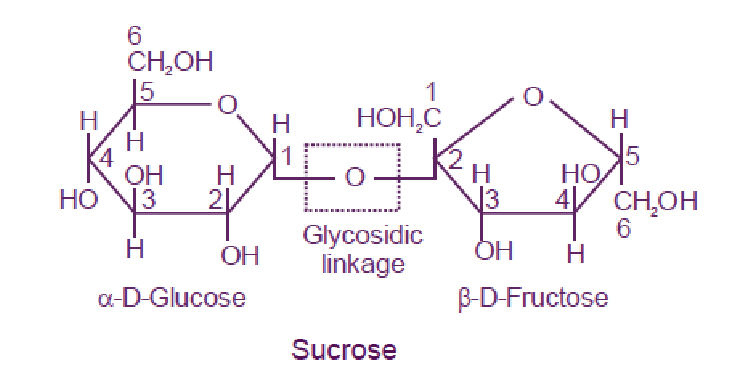Hence in sucrose glycosidic linkage between C1 of α-glucose and C2 of β-D-fructose is found

Maltose ⇒ Glycosidic linkage between C1 and C4

Lactose ⇒ Glycosidic linkage between C1 and C4

Amylose ⇒ Glycosidic linkage between C1 and C4

19. Some drugs bind to a site other than the active site of an enzyme. This site is known as

(A) non-active site

(B) allosteric site

(C) competitive site

(D) therapeutic site

Sol. Some drugs do not bind to the enzyme’s active site. These bind to a different site of the enzyme, which is called the allosteric site.

20. In base vs acid titration, at the endpoint methyl orange is present as

(A) quinonoid form

(B) heterocyclic form

(C) phenolic form

(D) benzenoid form

Sol.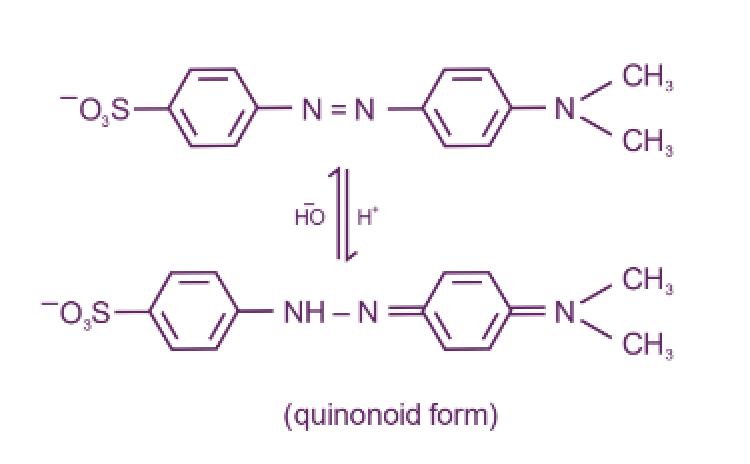Hence at the endpoint, methyl orange is present as quinonoid form.

SECTION – B

Numerical Value Type Questions: This section contains 10 questions. In Section B, attempt any five questions out of 10. The answer to each question is a NUMERICAL VALUE. For each question, enter the correct numerical value (in decimal notation, truncated/rounded-off to the second decimal place; e.g. 06.25, 07.00, –00.33, –00.30, 30.27, –27.30) using the mouse and the on-screen virtual numeric keypad in the place designated to enter the answer.

1. 56.0 L of nitrogen gas is mixed with excess of hydrogen gas and it is found that 20 L of ammonia gas is produced. The volume of unused nitrogen gas is found to be ____ L.

Sol. N2(g) + 3H2 (g) → 2NH3 (g)

Since H2 is in excess and 20 L of ammonia gas is produced.

Hence, 2 moles NH3 ≡ 1 mole N2 (v ∝ n)

20 L NH3 ≡ 10 L N2

Volume of N2 left = 56 – 10

= 46 L

2. A sealed flask with a capacity of 2 dm3 contains 11 g of propane gas. The flask is so weak that it will burst if the pressure becomes 2 MPa. The minimum temperature at which the flask will burst is _______ °C. [Nearest integer]

(Given: R = 8.3 JK–1 mol–1, Atomic masses of C and H are 12u and 1u, respectively.) (Assume that propane behaves as an ideal gas.)

Sol. From ideal gas equation,

PV = nRT

P = 2 × 106 Pa

V = 2 dm3 = 2 × 10–3 m3

R = 8.3 JK–1 mol–1

$$\begin{array}{l}n = \frac{11}{44}\text{ mol}\end{array}$$
$$\begin{array}{l}2 \times 10^6 \times 2 \times 10^{-3}=\frac{11}{44}\times 8.3 \times T\end{array}$$

T = 1927.7 K

T (in °C) = 1927.7 – 273 ≃ 1655 °C

3. When the excited electron of a H atom from n = 5 drops to the ground state, the maximum number of emission lines observed are ____.

Sol. Since there is a single hydrogen atom, so only

5 → 4, 4 → 3, 3 → 2, 2 → 1 lines are obtained.

4. While performing a thermodynamics experiment, a student made the following observations.

HCl + NaOH → NaCl + H2O; ΔH = – 57.3 kJ mol–1

CH3COOH + NaOH → CH3COONa + H2O; ΔH = –55.3 kJ mol–1

The enthalpy of ionization of CH3COOH, as calculated by the student, is ______ kJ mol–1. [nearest integer]

Sol. (I) HCl + NaOH → NaCl + H2O

ΔH1 = – 57.3 KJ mol–1

(II) CH3COOH + NaOH → CH3COONa + H2O

ΔH2 = – 55.3 KJ mol–1

Reaction (I) can be written as

(III) NaCl + H2O → HCl + NaOH

ΔH3 = 57.3 KJ mol–1

CH3COOH + NaCl → CH3COONa + HCl ΔHr

ΔHr = ΔH3 + ΔH2 = 57.3 – 55.3

= 2 kJ mol–1

5. For the decomposition of azomethane, CH3N2CH3(g) → CH3CH3(g) + N2(g), a first order reaction, the variation in partial pressure with time at 600 K is given as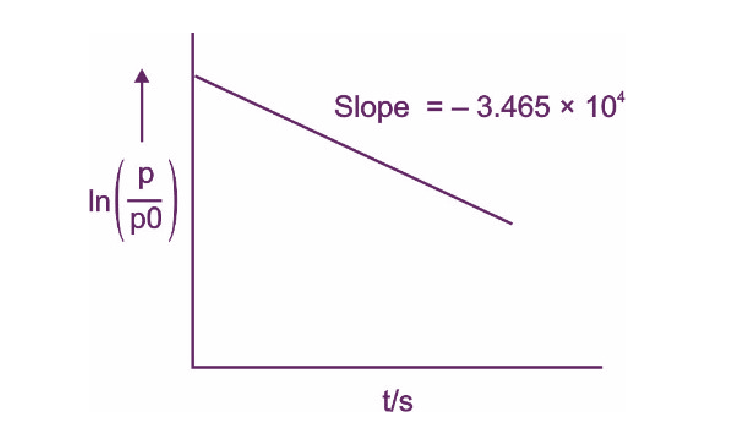The half life of the reaction is ______ × 10–5s. [Nearest integer]

Sol. For first order reaction,

ln A = ln A0 – kt

Hence Slope = –k

–k = –3.465 × 104

$$\begin{array}{l}k=\frac{0.693}{t_{1/2}}\end{array}$$
$$\begin{array}{l}3.465 \times 10^4 = \frac{0.693}{t_{1/2}}\end{array}$$

t1/2 = 2 × 10–5 s

6. The sum of number of lone pairs of electrons present on the central atoms of XeO3, XeOF4 and XeF6, is_____

Sol.

$$\begin{array}{l}XeO_3\Rightarrow S.N.\ (\text{Steric number})=\frac{1}{2}=4\Rightarrow sp^3\end{array}$$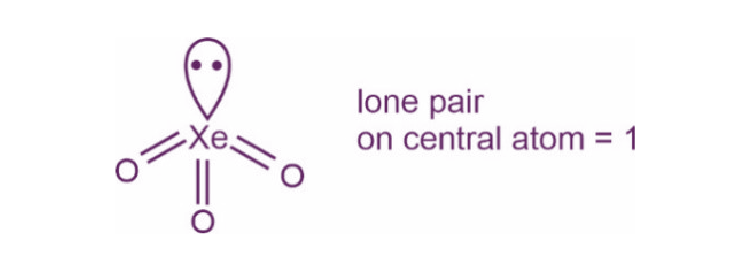$$\begin{array}{l}XeOF_4 \Rightarrow \text{ S.N } = \frac{1}{2}[8+4]=6 \Rightarrow sp^3d^2\end{array}$$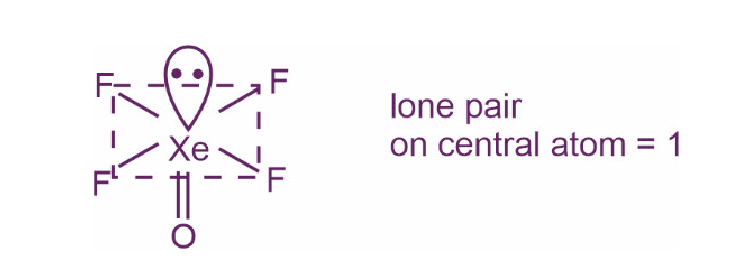$$\begin{array}{l}XeOF_6 \Rightarrow \text{ S.N } = \frac{1}{2}[8+6]=7 \Rightarrow sp^3d^3\end{array}$$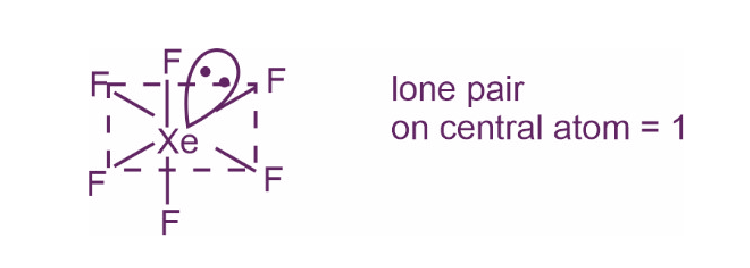Sum of lone pairs = 3

7. The spin-only magnetic moment value of M3+ ion (in gaseous state) from the pairs Cr3+/Cr2+, Mn3+/Mn2+, Fe3+/Fe2+ and Co3+/Co2+ that has negative standard electrode potential, is____ B.M. [Nearest integer]

Sol. Among the pairs given, Cr3+/Cr2+ has negative reduction potential which is –0.41 V.

Cr (III) ⇒ d3

Number of unpaired electrons = 3

$$\begin{array}{l}\mu = \sqrt{3(3+2)}=\sqrt{15} \simeq 4 \text{B.M.}\end{array}$$

8. A sample of 4.5 mg of an unknown monohydric alcohol, R–OH was added to methylmagnesium iodide. A gas is evolved and is collected and its volume measured to be 3.1 mL. The molecular weight of the unknown alcohol is___ g/mol. [Nearest integer]

Sol. R – OH + CH3 MgI ⇒ R – OMgI + CH4

moles of alcohol (ROH) ≡ moles of CH4

At STP, [Assuming STP]

1 mole corresponds to 22.7 L

$$\begin{array}{l}\text{Hence, 3.1 mL} \equiv \frac{3.1}{22700}\text{ mol}\end{array}$$

So, moles of alcohol

$$\begin{array}{l}=\frac{3.1}{22700}\end{array}$$
$$\begin{array}{l}\Rightarrow \frac{3.1}{22700}=\frac{4.5\times10^{-3}}{M}\end{array}$$
$$\begin{array}{l}\text{M}\simeq 33 \text{ g/mol}\end{array}$$

9. The separation of two coloured substances was done by paper chromatography. The distances travelled by solvent front, substance A and substance B from the base line are 3.25 cm, 2.08 cm and 1.05 cm, respectively. The ratio of Rf values of A to B is_____.

Sol.

$$\begin{array}{l}\text{R}_\text{f}=\frac{\text{Distance travelled by the substance}}{\text{Distace travelled by the solvent front}}\end{array}$$
$$\begin{array}{l}(R_f)_A=\frac{2.08}{3.25}\end{array}$$
$$\begin{array}{l}(R_f)_B=\frac{1.05}{3.25}\end{array}$$
$$\begin{array}{l}\frac{(R_f)_A}{(R_f)_B}\simeq 2\end{array}$$

10. The total number of monobromo derivatives formed by the alkanes with molecular formula C5H12 is (excluding stereo isomers)____.

Sol. Total monobromo derivatives = 8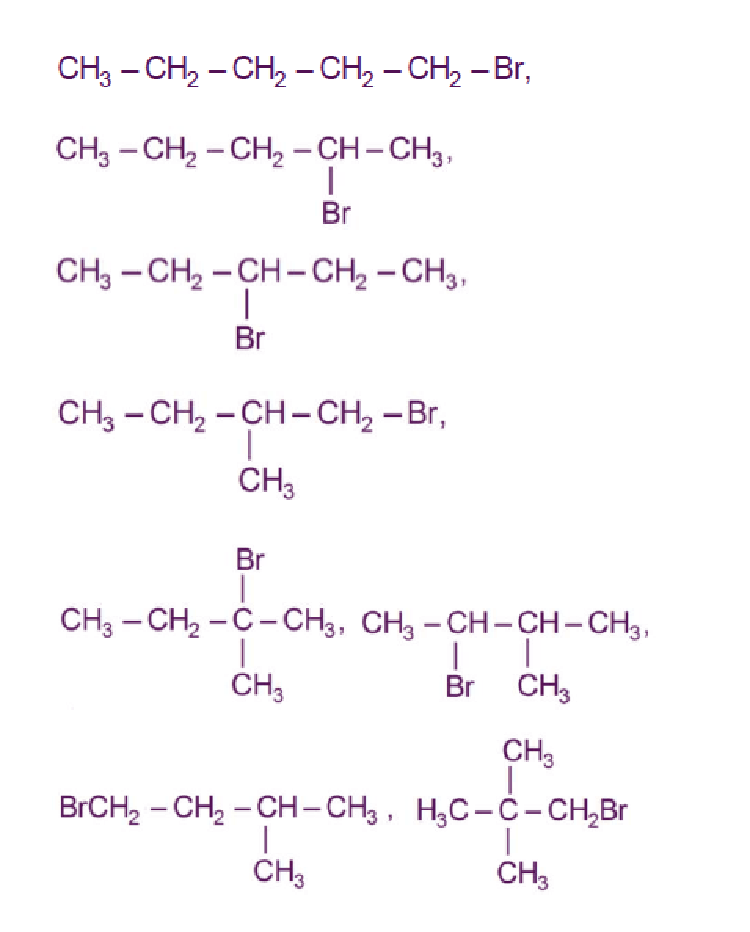### Download PDF of JEE Main 2022 July 25 Shift 2 Chemistry Paper & Solutions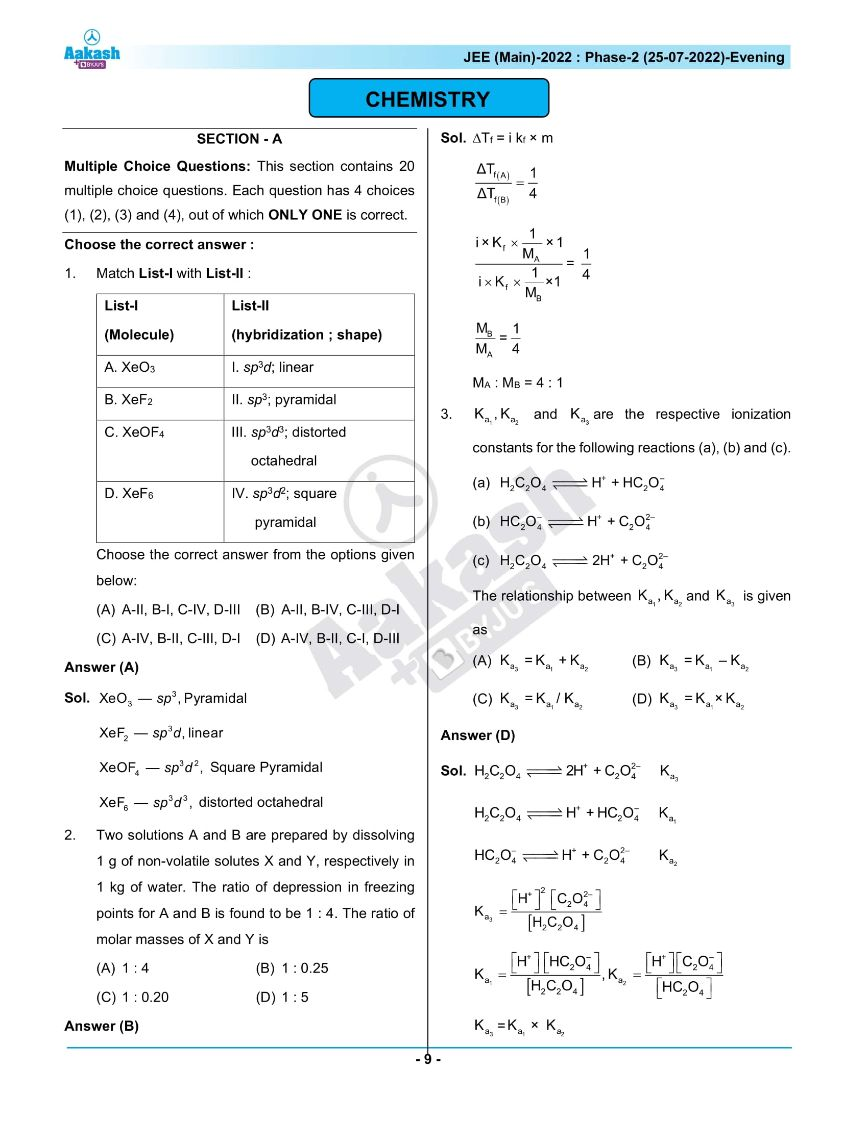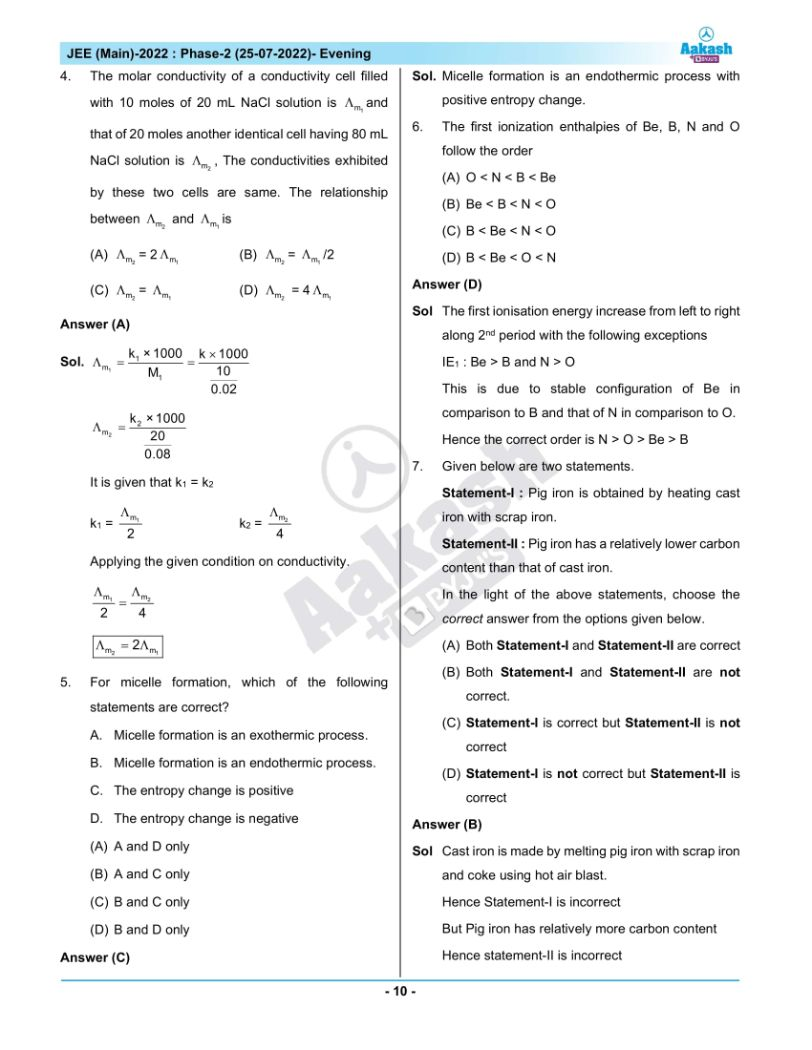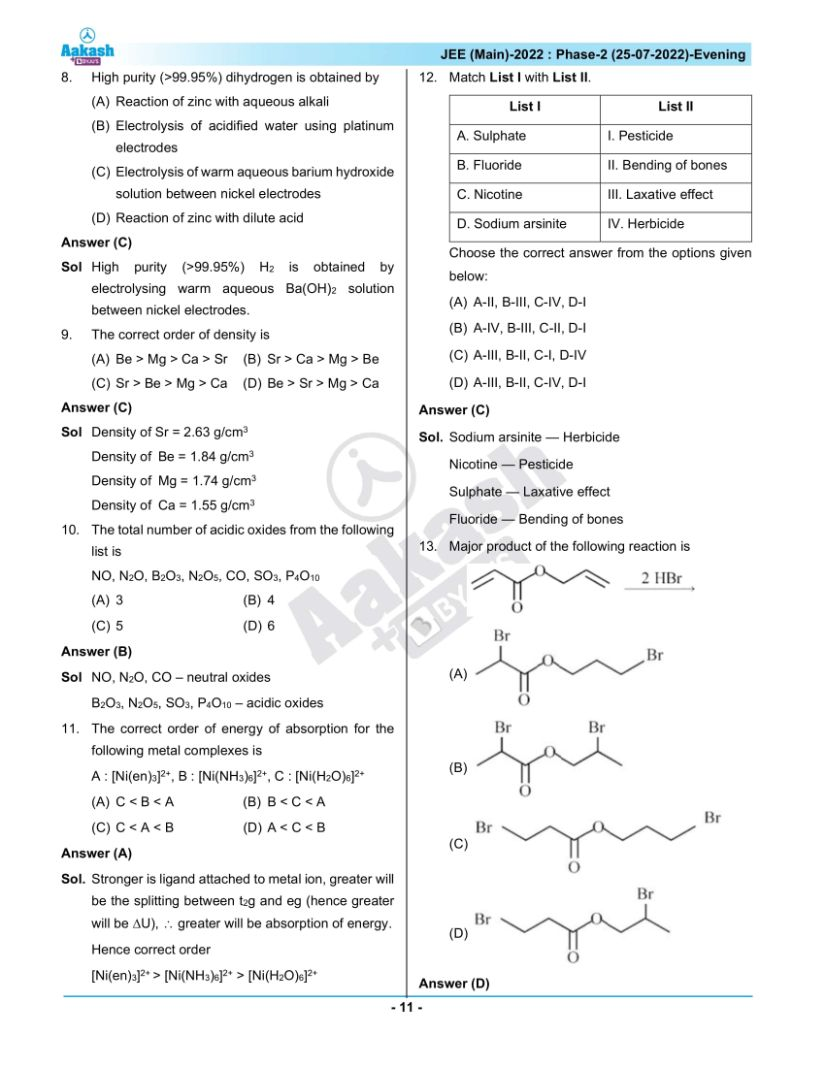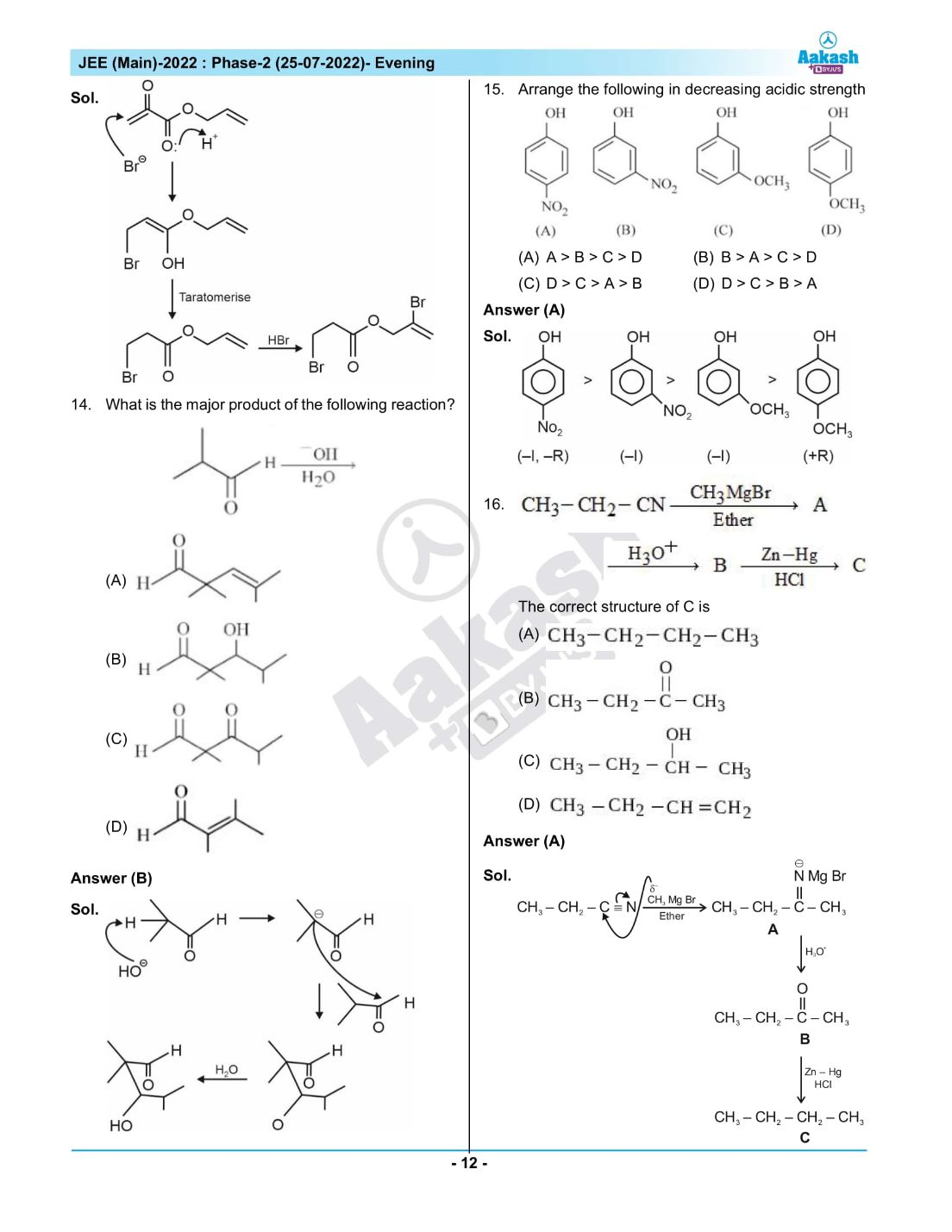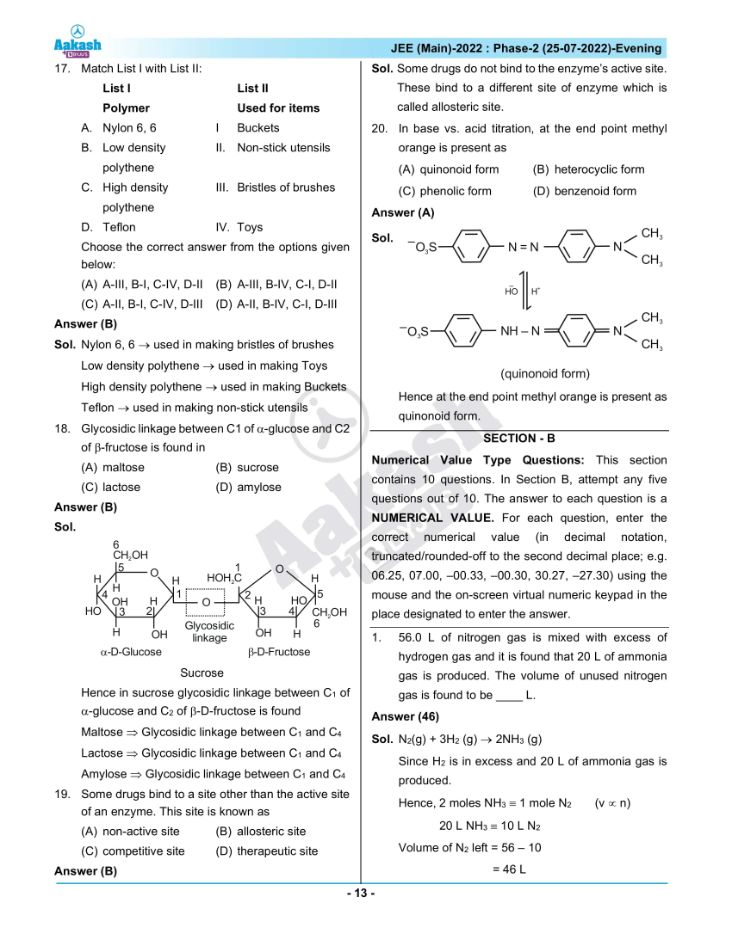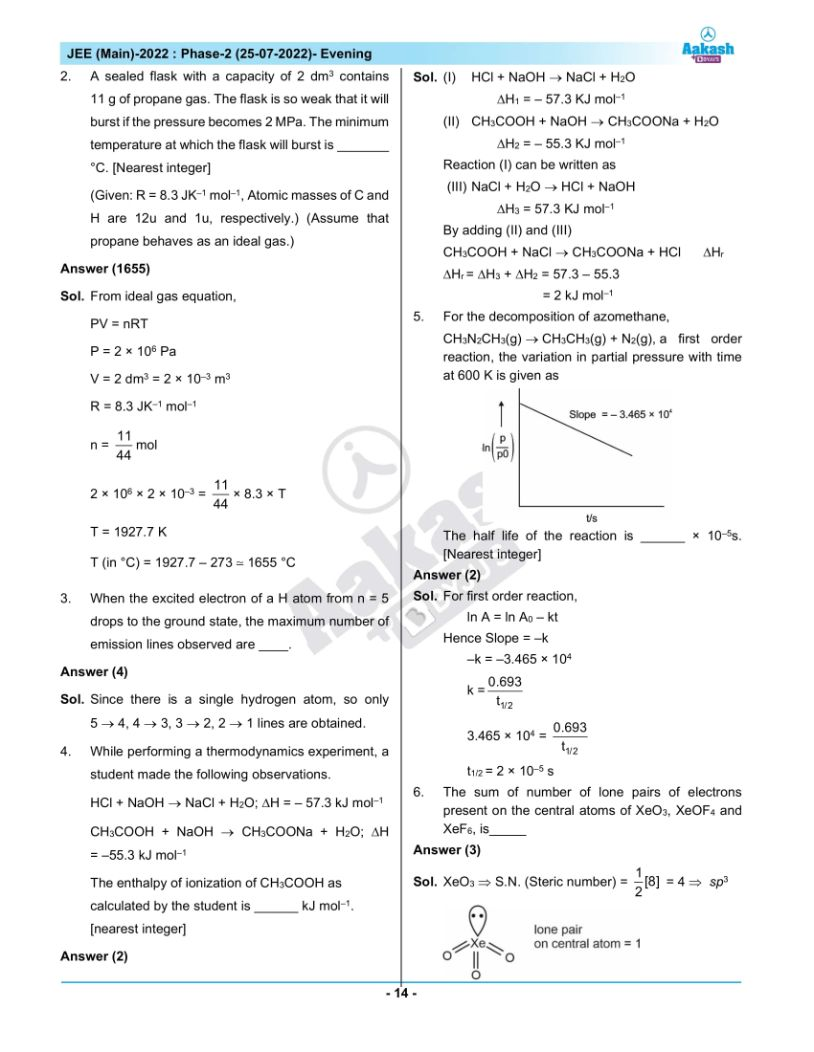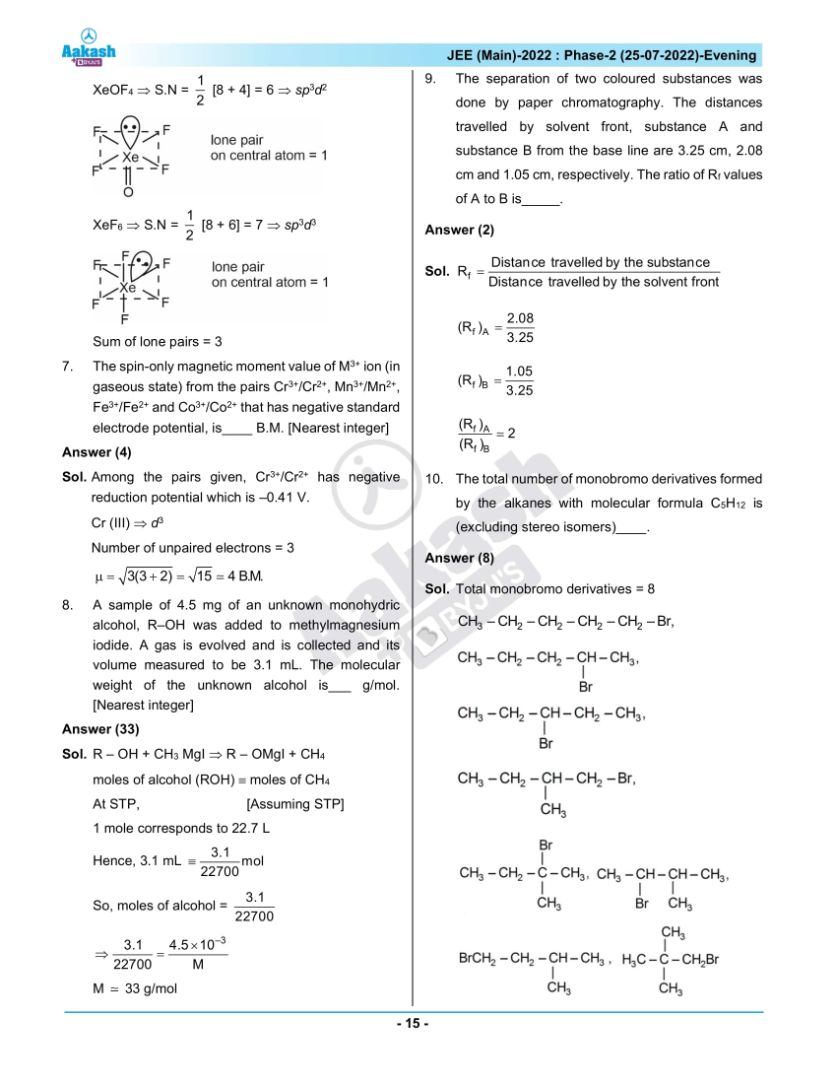## JEE Main 2022 July 25th Shift 2 Paper Analysis